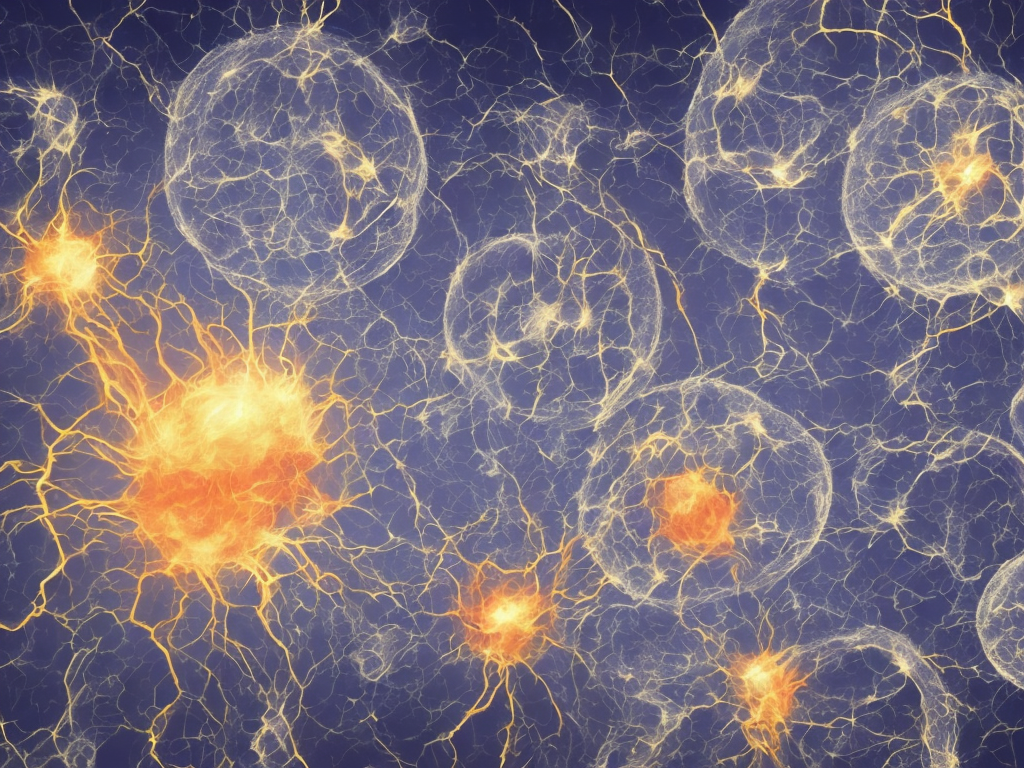# Explain The Difference Between Displacement And Double Displacement Reaction

tl;dr
Displacement reactions involve the exchange of an atom or group of atoms between two compounds, while double displacement reactions involve the exchange of ions between two compounds to form different compounds.Chemical reactions are fundamental processes that occur in our daily lives. They involve the rearrangement of atoms to form new substances with different properties. Displacement and double displacement reactions are two common types of chemical reactions that occur when substances interact with each other. While both these reactions involve the exchange of atoms or groups of atoms, they differ in terms of the number and types of substances involved. In this article, we will explore the difference between displacement and double displacement reactions.

Displacement reactions, also known as single replacement reactions, are chemical reactions in which an element or a compound displaces another element or compound from a chemical compound. In simpler terms, an element or a compound takes the place of another element or compound in a chemical reaction. The key characteristic of displacement reactions is that they involve the exchange of an atom or a group of atoms between two compounds, resulting in the formation of a new compound.

An example of a displacement reaction is the reaction between zinc metal (Zn) and hydrochloric acid (HCl) to form zinc chloride (ZnCl2) and hydrogen gas (H2). The chemical equation for this reaction can be written as:

Zn + 2HCl → ZnCl2 + H2

In this reaction, the zinc atom displaces the hydrogen atom in the hydrochloric acid to form zinc chloride and hydrogen gas. The reaction is represented by the arrow (→), indicating the direction of the reaction.

Another example of a displacement reaction is the reaction between magnesium metal (Mg) and copper sulfate (CuSO4) to form magnesium sulfate (MgSO4) and copper metal (Cu). The chemical equation for this reaction can be written as:

Mg + CuSO4 → MgSO4 + Cu

In this reaction, magnesium displaces copper from copper sulfate to form magnesium sulfate and copper metal. The displacement reaction results in a change of both physical and chemical properties of the compounds involved.

On the other hand, double displacement reactions, also known as double replacement reactions or metathesis reactions, involve the exchange of ions between two compounds to form different compounds. In these reactions, the positive ions in the reactants swap places with each other, resulting in the formation of new compounds.

An example of a double displacement reaction is the reaction between silver nitrate (AgNO3) and sodium chloride (NaCl) to form silver chloride (AgCl) and sodium nitrate (NaNO3). The chemical equation for this reaction can be written as:

AgNO3 + NaCl → AgCl + NaNO3

In this reaction, the positive ion Ag+ in silver nitrate swaps places with the positive ion Na+ in sodium chloride, resulting in the formation of silver chloride and sodium nitrate. The double displacement reaction occurs because the products formed are more stable than the reactants.

Another example of a double displacement reaction is the reaction between calcium hydroxide (Ca(OH)2) and sulfuric acid (H2SO4) to form calcium sulfate (CaSO4) and water (H2O). The chemical equation for this reaction can be written as:

Ca(OH)2 + H2SO4 → CaSO4 + 2H2O

In this reaction, the positive ion Ca2+ in calcium hydroxide swaps places with the positive ion H+ in sulfuric acid, resulting in the formation of calcium sulfate and water.

One key difference between displacement and double displacement reactions is the number of substances involved. In displacement reactions, only two substances are involved, whereas in double displacement reactions, there are typically four substances involved. Displacement reactions involve the exchange of an atom or group of atoms between two compounds, resulting in the formation of a new compound. On the other hand, double displacement reactions involve the exchange of ions between two compounds to form different compounds.

Another difference between the two types of reactions is the nature of the compounds involved. Displacement reactions often involve a metal reacting with another substance to form a new compound. For example, in the reaction between zinc metal and hydrochloric acid, zinc displaces hydrogen to form zinc chloride. On the other hand, double displacement reactions often involve ionic compounds, where the positive ions in the reactants swap places with each other to form new compounds. For example, in the reaction between silver nitrate and sodium chloride, the positive ions Ag+ and Na+ swap places to form silver chloride and sodium nitrate.

In summary, displacement and double displacement reactions are two common types of chemical reactions that occur when substances interact with each other. Displacement reactions involve the exchange of an atom or group of atoms between two compounds, resulting in the formation of a new compound. Double displacement reactions involve the exchange of ions between two compounds to form different compounds. While displacement reactions involve only two substances, double displacement reactions involve four substances. Understanding the differences between these reactions can help in predicting the products of chemical reactions and studying the properties of substances.